﻿ GATE CS Applied Course

## GATE Previous Subject Wise Questions

##### Question 1
A certain processor uses a fully associative cache of size 16 kB, The cache block size is 16 bytes. Assume that the main memory is byte addressable and uses a 32-bit address. How many bits are required for the Tag and the Index fields respectively in the addresses generated by the processor?
A
24 bits and 0 bits
B
28 bits and 4 bits
C
24 bits and 4 bits
D
28 bits and 0 bits
computer organization GATE 2019 Cache and main memory
##### Question 2
The chip select logic for a certain DRAM chip in a memory system design is shown below. Assume that the memory system has 16 address lines denoted by A15 to A0. What is the range of address (in hexadecimal) of the memory system that can get enabled by the chip select (CS) signal?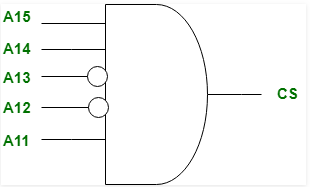A
C800 to CFFF
B
CA00 to CAFF
C
C800 to C8FF
D
DA00 to DFFF
computer organization GATE 2019
##### Question 3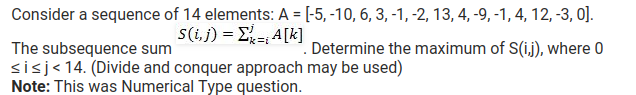A
29
B
19
C
39
D
09
GATE 2019
##### Question 4
There are n unsorted arrays: A1, A2, ....,An. Assume that n is odd. Each of A1, A2, ...., An contains n distinct elements. There are no common elements between any two arrays. The worst-case time complexity of computing the median of the medians of A1, A2, ....,An is ________ .
A
Ο(n log n)
B
Ο(n2)
C
Ο(n)
D
Ω(n2log n)
Algorithms GATE 2019
##### Question 5
Let G be any connection, weighted, undirected graph:
• I. G has a unique minimum spanning tree if no two edges of G have the same weight.
• II. G has a unique minimum spanning tree if, for every cut G, there is a unique minimum weight edge crossing the cut.
Which of the above two statements is/are TRUE?
A
Neither I nor II
B
I only
C
II only
D
Both I and II
Algorithms GATE 2019
##### Question 6
Consider the following statements:
• I. The smallest element in a max-heap is always at a leaf node.
• II. The second largest element in a max-heap is always a child of the root node.
• III. A max-heap can be constructed from a binary search tree in Θ(n) time.
• IV. A binary search tree can be constructed from a max-heap in Θ(n) time.
Which of the above statements is/are TRUE?
A
II, III and IV
B
I, II and III
C
I, III and IV
D
I, II and IV
Algorithms GATE 2019
##### Question 7
A certain processor deploys a single-level cache. The cache block size is 8 words and the word size is 4 bytes. The memory system uses a 60 MHz clock. To service a cache-miss, the memory controller first takes 1 cycle to accept the starting address of the block, it then takes 3 cycles to fetch all the eight words of the block, and finally transmits the words of the requested block at the rate of 1 word per cycle. The maximum bandwidth for the memory system when the program running on the processor issues a series of read operations is _________ × 106 bytes/sec.
Note: This was Numerical Type question.
A
160
B
145
C
172
D
124
computer organization GATE 2019
##### Question 8
Let T be a full binary tree with 8 leaves. (A full binary tree has every level full.) Suppose two leaves a and b of T are chosen uniformly and independently at random. The expected value of the distance between a and b in T (i.e., the number of edges in the unique path between a and b) is (rounded off to 2 decimal places) ___________ .
A
5.71 to 5.73
B
4.85 to 4.86
C
2.71 to 2.73
D
4.24 to 4.26
Data Structures GATE 2019
##### Question 9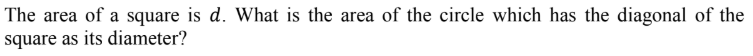AB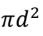C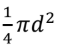Dgate 2018
##### Question 10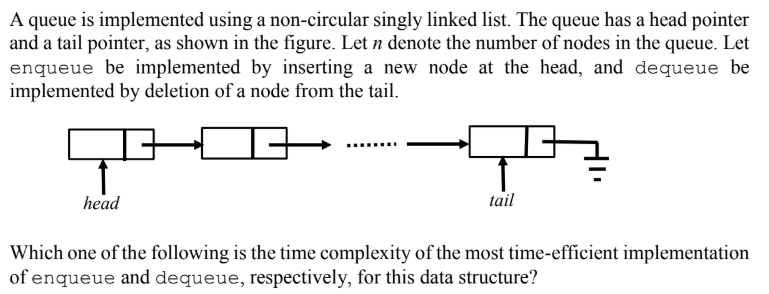A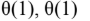B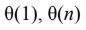CDData Structures gate 2018
##### Question 11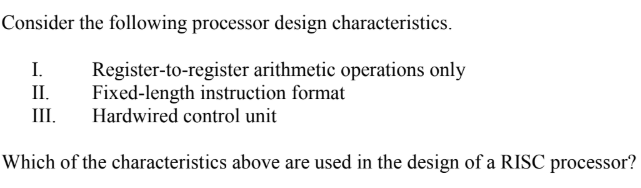A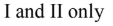B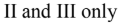CDcomputer organization gate 2018
##### Question 12Submit
Data Structures gate 2018
##### Question 13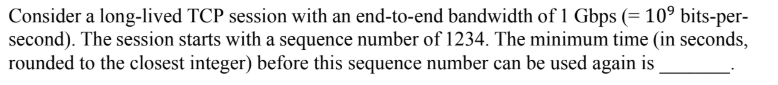Submit
Computer Networks gate 2018
##### Question 14A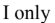B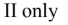C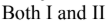DData Structures gate 2018 Graphs
##### Question 15A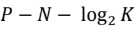B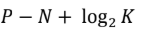C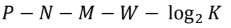D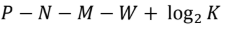computer organization gate 2018
##### Question 16Submit
Data Structures gate 2018 Heap-Tree
##### Question 17Submit
Algorithms gate 2018 Minimum-Spanning-Tree
##### Question 18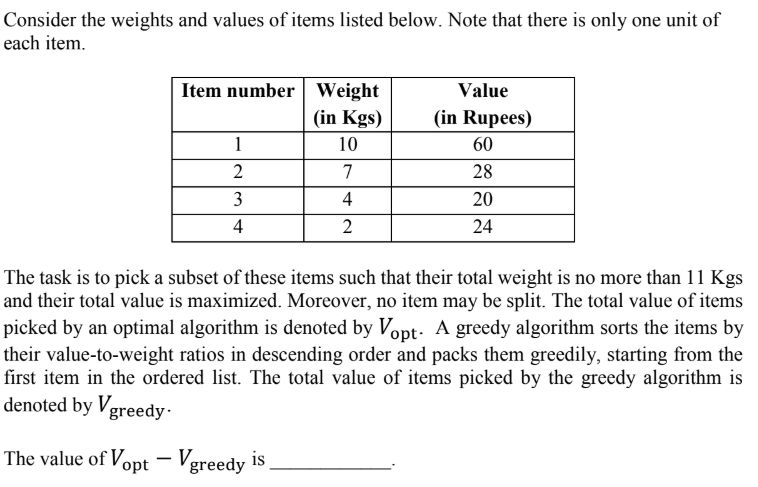Submit
Algorithms gate 2018
##### Question 19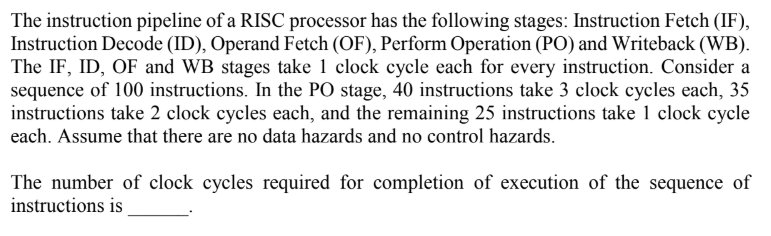Submit
computer organization gate 2018
##### Question 20Submit
computer organization gate 2018© 2023 - All rights are reserved- AAIC Technologies pvt ltd